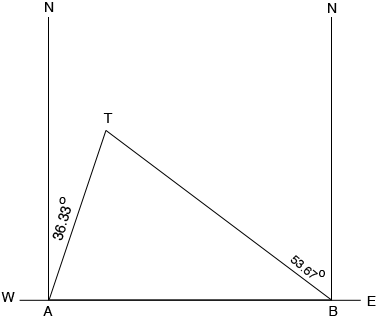SEARCH HOMEMath Central Quandaries & QueriesQuestion from Nathan, a student: Radio direction finders set up two points A and B, which are 2.5 miles apart on an east-west line. From A, it is found that the bearing of a signal from a radio transmitter is N36.33 degrees E, while from B the bearing of the same signal is N53.67 degrees W. Find the distance of the transmitter from B.Hi Nathan,

I drew a diagram.The transmitter is at $T.$

What is the measure of angle $TAB?$

What is the measure of angle $ABT?$

What is the measure of angle $BTA?$

PennyMath Central is supported by the University of Regina and The Pacific Institute for the Mathematical Sciences.